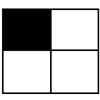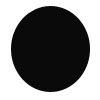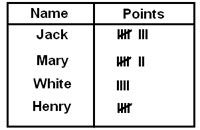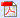## Aug 24, 2009

1. 500+220=_______

a. 400+20

b. 500+20

c. 600+20

d. 700+20

2. The place value of 2 in 324 is ____

a. 2 hundreds

b. 2 ones

c. 2 tens

d. 2 zeros

3. Choose an odd number from the given set of numbers

a. 654

b. 379

c. 128

d. 986

4. Choose a number which is greater than 979.

a. 901

b. 799

c. 999

d. 979

5. Which of the following number set is in ascending order?

a. 40, 400, 4, 0

b. 0, 40, 4, 400

c. 400, 4, 40, 0

d. 0, 4, 40, 400

6.The shaded portion of the picture represents _________

a. 1/3

b. ¼

c. ¾

d. 4/3

7. 205, 204, 203, 20, 201, 200, ______

a. 399

b. 299

c. 199

d. 99

8. Which of the following number should be added with 2 to get 14?

a. 2

b. 8

c. 12

d. 18

9. Richard got 12 dollars from mom on first day and 19 dollars on second day. He gave 7 dollars to his friend on third day. Find the amount left with Richard.

a. 5dollars

b. 12 dollars

c. 24 dollars

d. 31dollars

10. 3+(4+5)=___

a. (3+4)+5

b. 3-(4+5)

c. 4+5

d. 3+4

11. Fill in the appropriate sign:

12 ___ 2 = 6

a. +

b. ÷

c. ×

d. %

12. Each day Christina eats 3 candies. How many candies did she eat in 11 days?

a. 8

b. 14

c. 33

d. 50

13. Fill in the missing symbol.

∆, ↑, ◊, ∆, ↑, ◊, ∆, ↑, ◊, ?

a.

b.

c.

d.

14. Find the missing number.

11, 21, 31, 41, ____, 61, 71, 81.

a. 43

b. 46

c. 49

d. 51

15. 15 - ? =6

a. 6

b. 7

c. 8

d. 9

16.The given figure is a ______

a. Square

b. Rectangle

c. Circle

d. pentagon

17. 60 minutes=________

a. 1 hour

b. 1 second

c. 1 day

d. 1 month

18. 1 dollar=________

a. 3 quarters

b. 4 quarters

c. 5 quarters

d. 6 quarters

19. Four students from second grade take test in math worksheet. Find the points scored by Mary.a. 7

b. 6

c. 5

d. 4

20. Weight of an object can be represented in ______

a. Ounces

b. Miles

c. Pints

d. hours

Questions: